## Known Design Methods

Let us briefly recall some of the known methods used to design space-time precoders.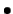Design based on pairwise error probability: this is the well-known criterion of maximizing the rank and the determinant of the difference matrix . Algebraic methods were used to design space-time codes that are full-rate , i.e. one symbol per transmit antenna per symbol time. Such algebraic space-time precoders have been optimized for uncoded linear modulations under maximum-likelihood detection. Some of them do not exist for all values of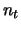. Furthermore, all precoders designed by this criterion for the quasi-static channel have been developed with full-spreading where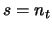. As an example, the Golden code  is an algebraic precoder optimized for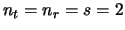, its precoding matrix is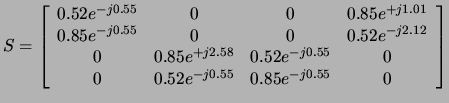(2)

The weak point in constructing rotations via the pairwise error probability criterion is the fact that error-correcting codes that may be serially concatenated with the precoder are not taken into account by the design criterion.Design maximizing the ergodic channel capacity: linear dispersion (LD) codes  are designed for multiple antenna channels by a search that maximizes the ergodic capacity under a gaussian channel input. Such a design is not necessarily suitable for a block fading channel with a finite number of states, e.g.,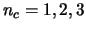, etc. Also, the kind of input alphabet is not considered in the search for linear dispersion codes.Design based on genie conditions: this construction was introduced in  where perfect a priori probability feedback is assumed in the iterative joint detection and decoding of ST-BICM. In order to guarantee maximum diversity order and maximum coding gain at the output of the detector, the design must guarantee two conditions: 1- Orthogonal sub-rows in the linear precoding matrix, and 2- Equal norm sub-rows in the linear precoding matrix. In ST-BICM, there exists a strong interaction between the error correcting code with interleaving and the linear precoder, both in terms of diversity and coding gain maximization . Complexity can be controlled by the choice of a minimal spreading factor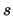that guarantees full diversity . The genie conditions are optimal, in terms of ML performance, when all diversity given by the transmit antennas is collected at the detector (i.e.). A supplementary condition called Dispersive Nucleo Algebraic'' (DNA) has been proposed in  to keep optimality when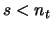while having the genie conditions on sub-groups of transmit antennas.
As an example, the cyclotomic rotation given below is an algebraic precoder satisfying the genie conditions for ST-BICM with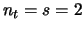: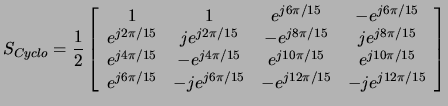(3)

Consider the space-time bit-interleaved coded modulation drawn in Fig. 1. This ST-BICM is a serial concatenation of a rate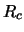binary convolutional code, an interleaver of size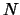bits, and a QAM mapper followed by the precoder as described in the previous section. When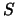is the identity matrix, the ST-BICM diversity order is upper-bounded by :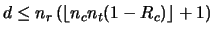(4)

The maximal diversity given by the outage limit under a finite size QAM alphabet also achieves the above Singleton bound .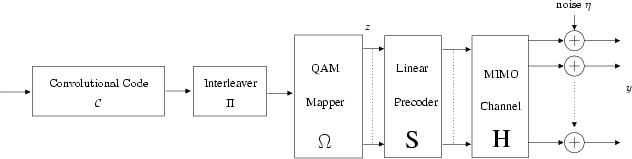With a vanishing coding rate, i.e.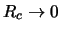, it is possible to attain the overall system diversity order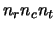produced by the receive antennas, the transmit antennas and the distinct channel states. Unfortunately, this is unacceptable due to the vanishing transmitted information rate. Precoding is one means to achieve maximum diversity with a non-vanishing coding rate. Under linear precoding that spreads QAM symbols overtime periods, the Singleton bound becomes :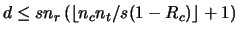(5)

Now if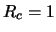, from the above inequality, we observe that precoding may achieve maximal diversity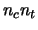without the use of error-correcting codes. Unfortunately, near outage performance is impossible in this case due to the weak coding gain of all kinds of precoders. The near-outage performance of ST-BICM is a judicious trade-off between error-control coding and linear QAM precoding. Hence, we propose a simple information theoretical design of multi-dimensional rotations that take into account the interaction between channel coding and symbol space-time spreading.

Joseph Jean Boutros 2005-05-07